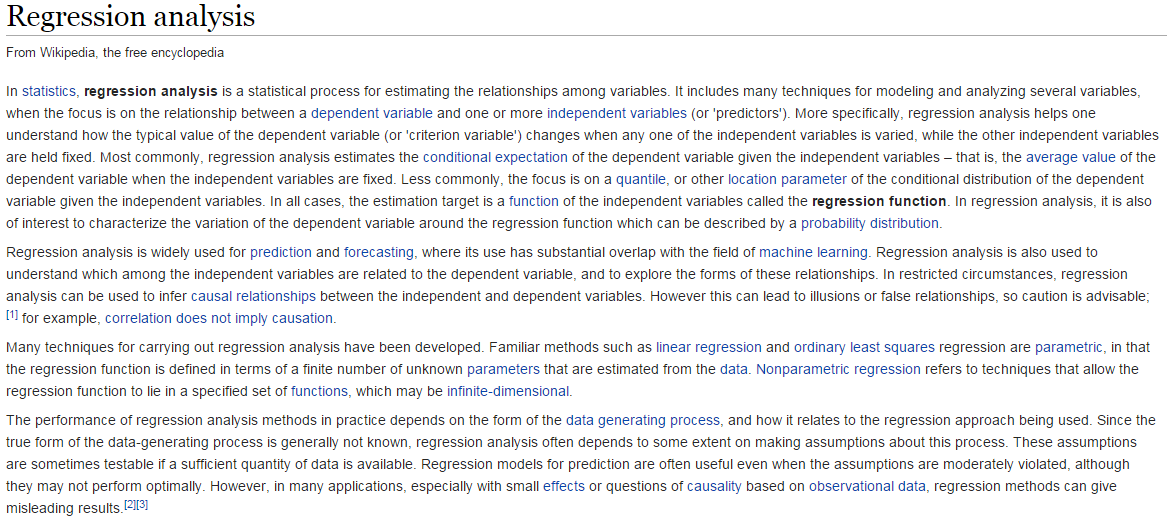# 0 – Brief Introduction to Regression Analysis

 Section 0 – Brief Introduction to Regression Analysis STAT 360 – Regression Analysis Fall 2017 0 – Brief Introduction to Regression Analysis According to the source of all knowledge - - Wikipedia (tongue placed firmly in cheek)Regression in general refers to the process of estimating conditional expectations (i.e. means) and variances of a given outcome of interest. In regression one variable is viewed as the response (Y) and the other variables are predictors (X’s). We wish understand how the mean of the response varies as function of the predictors, or more generally how Y relates to or is associated with the predictors. In my opinion about 90% of what we do in statistics can be framed as a regression analysis problem, thus it is an EXTREMELY important topic in statistics. For example, t-tests (one- and two-sample), one-way ANOVA, etc. can all be put into the context of a regression analysis as we will see later. Here are some examples of questions where regression analysis could be employed to help answer them. How is the length of smallmouth bass in West Bearskin Lake related to their age in years and the radius of their scales in mm? How does the mean selling price of a home in Saratoga, NY relate to the physical features of a home like the # of bedrooms, # of bathrooms, square feet of living space, whether the home has a fireplace or not, etc.? Can explain how price relates to these characteristics? Can we accurately predict what a home will sell for given these characteristics? (Links: www.zillow.com and www.redfin.com) How does the dose of drug relate to the forced expiratory volume of asthmatic patients taking it? Do the dose levels differ? If so, by how much? Does changing class size affect success of students? Do changes in diet result in changes in cholesterol level, and if so, do the results depend on other characteristics such as age, gender, and amount of exercise? Do conservation easements on agricultural property, such as those passed recently regarding watershed buffers in MN, lower land value? Does having a right heart catheter used during treatment of heart attack patients increase 30-day mortality? After adjusting for demographics, what maternal risk factors are related to the birth weight (g) of infants or their chance of having an infant prematurely (< 37 weeks gestation)? What is the relationship between teacher salaries and district/county characteristics in MN? How are student basic skills test scores related to these factors? How are the shape and size of breast tumor cells related to the malignancy? Are cells spherical/circular and does the answer depend on malignancy status of the cells? Can model the probability that a cell is malignant (or benign) based on these measurements? How is the survival time of lung cancer patients related to the form of treatment they receive taking other factors into account? The possibilities are infinite! In this course we will primarily be focusing on regression situations where the response (Y) is a numeric variable. For a numeric response we typically use linear regression. However, we will also be discussing logistic regression where the response is a dichotomous (2 levels) categorical/nominal variable (i.e. “yes? vs. “no” or “success” vs. “failure”). Before we begin discussing linear regression in earnest however we will begin “reviewing”statistical preliminaries, e.g. descriptive statistics (both numeric & visual summaries), hypothesis/significance tests, confidence intervals, etc. in Section 1. Additional Notes: Share with your friends: